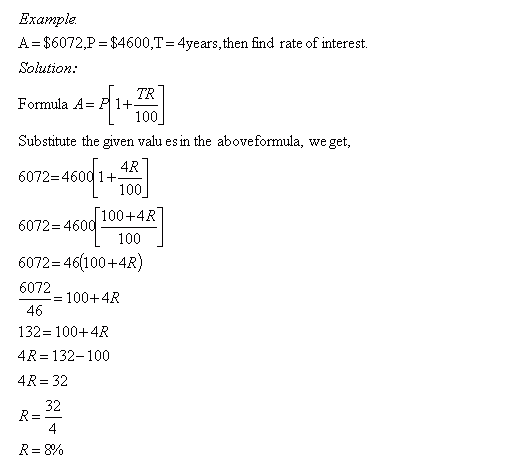Name: ___________________Date:___________________

 Email us to get an instant 20% discount on highly effective K-12 Math & English kwizNET Programs!

Grade 8 - Mathematics9.32 Simple Interest - VIIIDirections: Solve the following problems. Also write at least ten examples of your own.Name: ___________________Date:___________________

Grade 8 - Mathematics9.32 Simple Interest - VIII

 Q 1: A = \$1725, P = \$1500 and T = 3years, then find rate of interest.2.5%2%6%5% Q 2: A = \$2950, P = \$2500 and T = 3years, then find rate of interest.5%3%5.5%6% Q 3: A = \$10230, P = \$9300 and T = 3years, then find rate of interest.1.5%2.5%2%1% Q 4: A = \$8284, P = \$7600 and T = 3years, then find rate of interest.3%4%3.5%2.5% Q 5: A = \$3556, P = \$2800 and T = 3years, then find rate of interest.8%3%6%9% Q 6: A = \$5428, P = \$4600 and T = 2years, then find rate of interest.6%9%8%5% Question 7: This question is available to subscribers only! Question 8: This question is available to subscribers only!